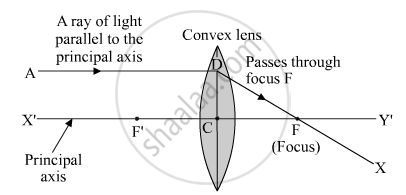Share

# Draw the Given Diagram in Your Answer Book and Complete It for the Path of a Ray of Light After Passing Through the Lens. - CBSE Class 10 - Science

ConceptRepresentation of Images Formed by Spherical Mirrors Using Ray Diagrams - Convex Mirror

#### Question

Draw the given diagram in your answer book and complete it for the path of a ray of light after passing through the lens.

#### Solution

The ray will pass through the focus after refraction since it is parallel to the principle axis. See the diagram given below.Is there an error in this question or solution?

#### APPEARS IN

Solution Draw the Given Diagram in Your Answer Book and Complete It for the Path of a Ray of Light After Passing Through the Lens. Concept: Representation of Images Formed by Spherical Mirrors Using Ray Diagrams - Convex Mirror.
S